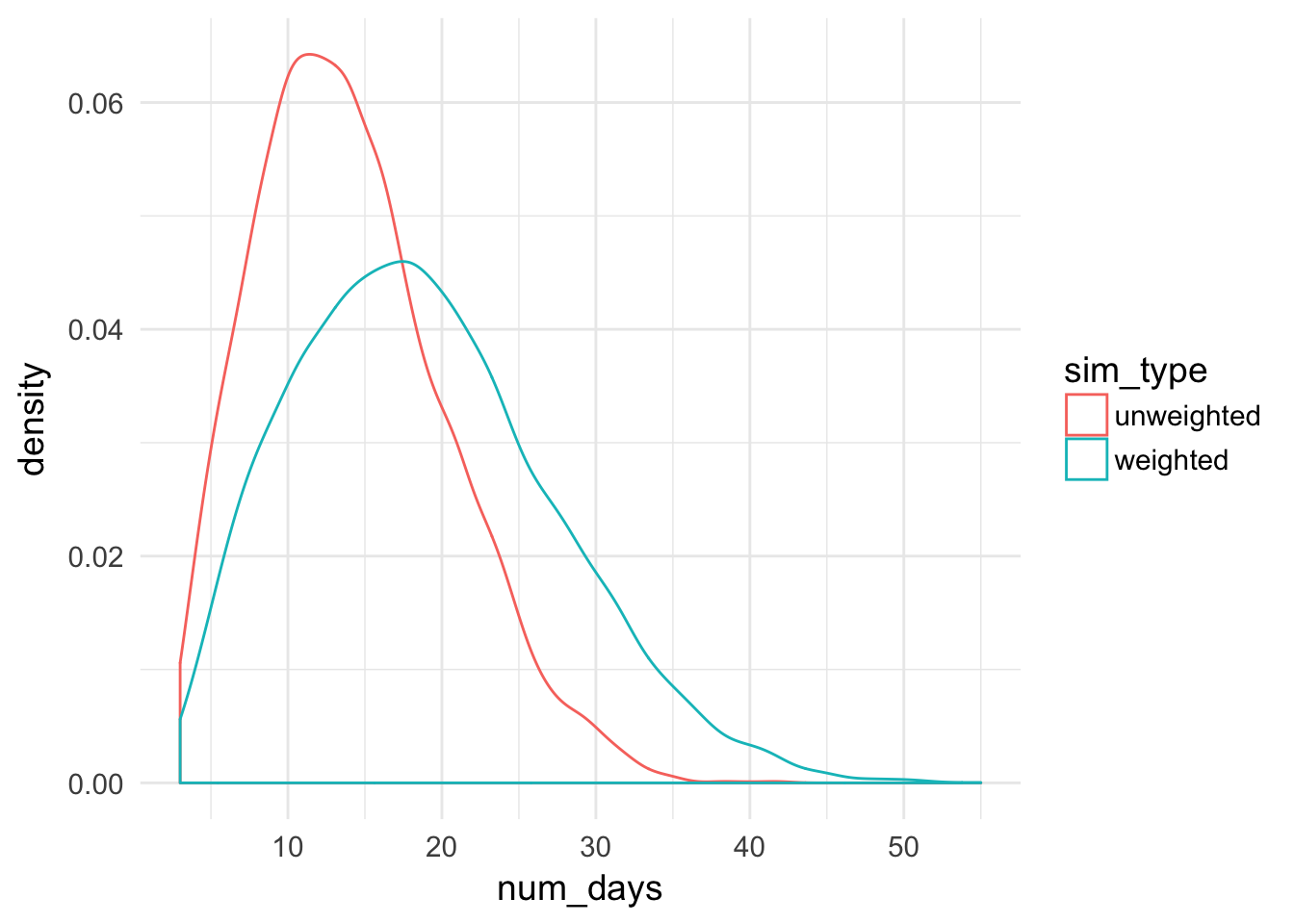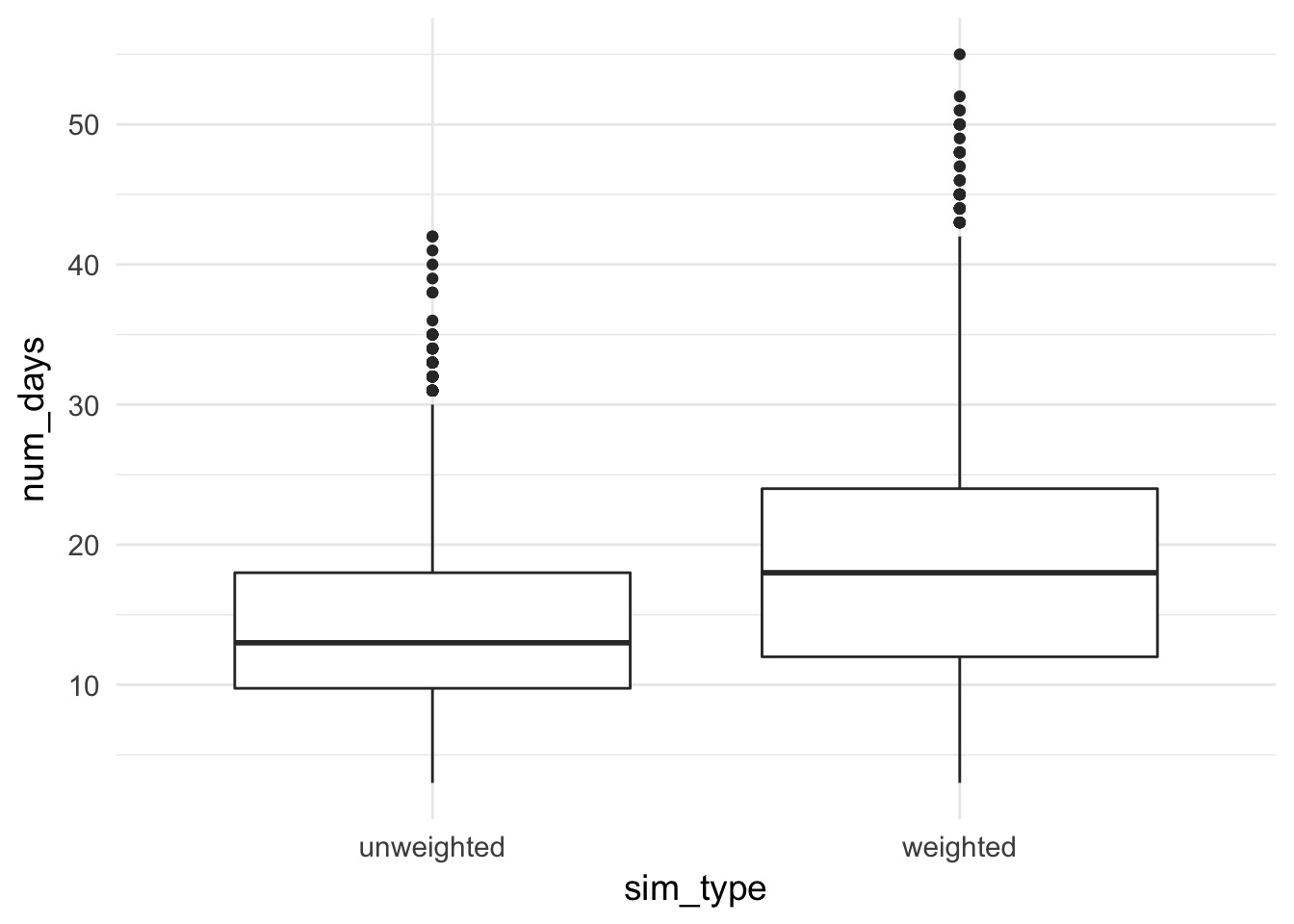### Solution to 538’s aug25/2017 Riddler Express

##### timothy leffel (@lefft)

here is the problem (original post from 538 here):

You take half of a vitamin every morning. The vitamins are sold in a bottle of 100 (whole) tablets, so at first you have to cut the tablets in half. Every day you randomly pull one thing from the bottle — if it’s a whole tablet, you cut it in half and put the leftover half back in the bottle. If it’s a half-tablet, you take the vitamin. You just bought a fresh bottle. How many days, on average, will it be before you pull a half-tablet out of the bottle?

Extra credit: What if the halves are less likely to come up than the full tablets? They are smaller, after all.

note: extra credit is the case where weighted=TRUE

#### 1. draw a pill from our bottle, with or without weights.

• weighted=TRUE means that full pills are inherently twice as likely to be drawn as half pills
• weighted=FALSE means that full pills and half pills have the same inherent likelihood of being drawn
draw_pill <- function(bottle, weighted){
if (!weighted){
weight_full <- sum(bottle=="full_pill")
weight_half <- sum(bottle=="half_pill")
weights <- c(weight_full, weight_half) / (weight_full + weight_half)
} else {
weight_full <- sum(bottle=="full_pill") * 2
weight_half <- sum(bottle=="half_pill")
weights <- c(weight_full, weight_half) / (weight_full + weight_half)
}
return(sample(c("full_pill", "half_pill"), size=1, prob=weights))
}

#### 2. after drawing a pill, update the bottle

• if you draw a full, put a half back in the bottle
• if you draw a half, just remove the half
update_bottle <- function(bottle, draw, day_num){
full_pills_left <- sum(bottle=="full_pill")
half_pills_left <- sum(bottle=="half_pill")

if (draw=="full_pill"){
bottle <- c(rep("full_pill", times=full_pills_left-1),
rep("half_pill", times=half_pills_left+1))
return(bottle)
}
if (draw=="half_pill"){
bottle <- c(rep("full_pill", times=full_pills_left),
rep("half_pill", times=half_pills_left-1))
return(bottle)
}
}

#### 3. simulate pill-taking

• until you draw a half, then stop.
• return the number of days it took.
simulate_pill_taking <- function(weighted){
my_bottle <- rep("full_pill", times=100)
day_num <- 1
draw <- draw_pill(my_bottle, weighted=weighted)
while (draw != "half_pill"){
draw <- draw_pill(my_bottle, weighted=weighted)
my_bottle <- update_bottle(bottle=my_bottle, draw=draw, day_num=day_num)
day_num <- day_num + 1
}
# uncomment if you want to see how many fulls are left after day day_num
# message(paste0(sum(my_bottle=="full_pill"), " full pills left"))
return(day_num)
}

#### 4. run the simulation for weighted and unweighted, show results

• 10000 times apiece
• display the average number of days it takes
• then plot the results
num_sims <- 10000

# 10000 simulations, without weights
days_till_half_unweighted <-
replicate(n=num_sims, simulate_pill_taking(weighted=FALSE))

# 10000 simulations, with weights
days_till_half_weighted <-
replicate(n=num_sims, simulate_pill_taking(weighted=TRUE))

# collect the results
sim_data <- data.frame(
num_days = c(days_till_half_weighted, days_till_half_unweighted),
sim_type = rep(c("weighted", "unweighted"), each=num_sims)
)

here’s the averages:

message(paste0(
"if we're equally likely to draw a full or a half, it takes an average of ",
round(mean(days_till_half_unweighted), 1), " days to draw a half pill"
))
## if we're equally likely to draw a full or a half, it takes an average of 14.1 days to draw a half pill
message(paste0(
"if we're twice as likely to draw a full as a half, it takes an average of ",
round(mean(days_till_half_weighted), 1), " days to draw a half pill"
))
## if we're twice as likely to draw a full as a half, it takes an average of 18.8 days to draw a half pill

here’s a density plot showing the distribution of days it takes to get a half pill, with and without weights.

ggplot(sim_data, aes(color=sim_type, x=num_days)) + geom_density()aaaand a boxplot too, for the types who appreciate straining their eyes/minds.

ggplot(sim_data, aes(x=sim_type, y=num_days)) + geom_boxplot()